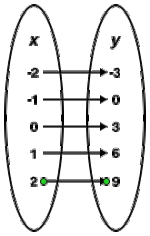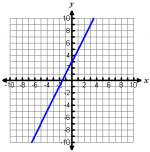# Let's Get Started

Let's explore the different ways to represent a function. Each representation of a function gives you certain information about the relationship between your input and output.

TEKS Standards and Student Expectations

A(12) Number and algebraic methods. The student applies the mathematical process standards and algebraic methods to write, solve, analyze, and evaluate equations, relations, and functions. The student is expected to:

A(12)(A) decide whether relations represented verbally, tabularly, graphically, and symbolically define a function

Resource Objective(s)

Represent a linear function in multiple ways.

Essential Questions

What is a function?

What are the different ways to represent a function?

What information do a table, graph, and equation give you?

Vocabulary

# Different Ways to Represent Functions

A mathematical relationship is how certain numbers (or objects) are connected to other numbers (or objects). In math, a relationship is a collection of ordered pairs (x, y).

A mathematical function is a special relationship where every input, x, has its own unique output, y.

A linear function is a polynomial function of degree zero or one and a relationship that has a graph that makes a straight line.

Relationships and functions can be represented as graphs, tables, equations, or verbal descriptions. Each representation gives us certain information.

Table of Values

A table of values, mapping diagram, or set of ordered pairs gives us a list of input values and their corresponding output values.

Example
Given = 3x - 2, the table, mapping diagram, and set of ordered pairs are shown.

Table of Values
 x Process y -2 3(-2) + 3 -3 -1 3(-1) + 3 0 0 3(0) + 3 3 1 3(1) + 3 6 2 3(2) + 3 9

Mapping DiagramSet of Ordered Pairs

(-2, 3), (-1, 0), (0, 3), (1, 6), (2, 9)

Graph

This representation gives us a a visual of the relationship. When given a graph, we can quickly determine if a relationship is a function, and what function family (if any) the relationship falls under.

Example:Equation

This representation gives us the rule on how to turn our input, x, into our output, y.

Example:

= 3x - 2. This tells us that to calculate our output, y, we have to multiply our input, x, by 3 and subtract 2.

Verbal Description

This representation gives us a way to create an application scenario for our relationship.

Example:

Mya's cell phone plan charges a monthly fee of \$39.95 for unlimited calling. There is an additional charge of .20 for each text message sent or received.